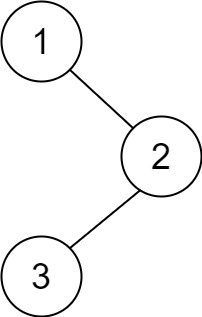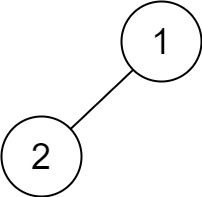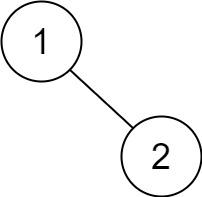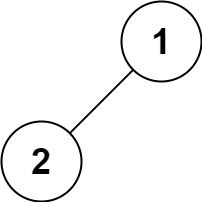## C# || How To Multiply Two Strings Using C#The following is a module with functions which demonstrates how to multiply two strings together using C#.

1. Multiply Strings – Problem Statement

Given two non-negative integers num1 and num2 represented as strings, return the product of num1 and num2, also represented as a string.

Note: You must not use any built-in BigInteger library or convert the inputs to integer directly.

Example 1:

``` Input: num1 = "2", num2 = "3" Output: "6" ```

Example 2:

``` Input: num1 = "123", num2 = "456" Output: "56088" ```

2. Multiply Strings – Solution

The following is a solution which demonstrates how to multiply two strings together.

This solution starts from the end of both strings and multiplies each individual number, keeping track of any carryovers. The result of each operation is stored in a ‘solution’ array, and when the operation is complete, the result is returned as a string.

``` 2. Multiply Strings - Solution C# // ============================================================================ // Author: Kenneth Perkins // Date: Oct 13, 2021 // Taken From: http://programmingnotes.org/ // File: Solution.cs // Description: Demonstrates how to multiply two strings together // ============================================================================ public class Solution { public string Multiply(string num1, string num2) { if (num1 == "0" || num2 == "0") { return "0"; } // This will be the max number of digits var solution = new int[num1.Length + num2.Length]; // Loop through both strings from the end for (int num1Index = num1.Length -1; num1Index >= 0; --num1Index) { for (int num2Index = num2.Length -1; num2Index >= 0; --num2Index) { // Get the current solution index var currentIndex = num1Index + num2Index + 1; // Multiply the two numbers var value = CharToInt(num1[num1Index]) * CharToInt(num2[num2Index]); // Add the value to the result at the current index solution[currentIndex] += value; // Check to see if there is any carry to be removed if (solution[currentIndex] > 9) { // Add the carry to the 'previous' index var carry = solution[currentIndex] / 10; solution[currentIndex - 1] += carry; // Remove the carry from the current index var digit = solution[currentIndex] % 10; solution[currentIndex] = digit; } } } // Skip over leading zeroes var zeroIndex = 0; while (zeroIndex < solution.Length && solution[zeroIndex] == 0) { ++zeroIndex; } // Convert to string and return var result = new StringBuilder(); for (int index = zeroIndex; index < solution.Length; ++index) { result.Append(solution[index]); } return result.ToString(); } public int CharToInt(char ch) { return ch - '0'; } }// http://programmingnotes.org/ 12345678910111213141516171819202122232425262728293031323334353637383940414243444546474849505152535455565758596061 // ============================================================================//    Author: Kenneth Perkins//    Date:   Oct 13, 2021//    Taken From: http://programmingnotes.org///    File:  Solution.cs//    Description: Demonstrates how to multiply two strings together// ============================================================================public class Solution {    public string Multiply(string num1, string num2) {        if (num1 ==  "0" || num2 == "0") {            return "0";        }         // This will be the max number of digits        var solution = new int[num1.Length + num2.Length];         // Loop through both strings from the end        for (int num1Index = num1.Length -1; num1Index >= 0; --num1Index) {            for (int num2Index = num2.Length -1; num2Index >= 0; --num2Index) {                // Get the current solution index                var currentIndex = num1Index + num2Index + 1;                 // Multiply the two numbers                var value = CharToInt(num1[num1Index]) * CharToInt(num2[num2Index]);                 // Add the value to the result at the current index                solution[currentIndex] += value;                 // Check to see if there is any carry to be removed                if (solution[currentIndex] > 9) {                     // Add the carry to the 'previous' index                    var carry = solution[currentIndex] / 10;                    solution[currentIndex - 1] += carry;                     // Remove the carry from the current index                    var digit = solution[currentIndex] % 10;                    solution[currentIndex] = digit;                }            }        }         // Skip over leading zeroes        var zeroIndex = 0;        while (zeroIndex < solution.Length && solution[zeroIndex] == 0) {            ++zeroIndex;        }         // Convert to string and return        var result = new StringBuilder();        for (int index = zeroIndex; index < solution.Length; ++index) {            result.Append(solution[index]);        }         return result.ToString();    }     public int CharToInt(char ch) {        return ch - '0';    }}// http://programmingnotes.org/ ```

QUICK NOTES:
The highlighted lines are sections of interest to look out for.

The code is heavily commented, so no further insight is necessary. If you have any questions, feel free to leave a comment below.

Once compiled, you should get this as your output for the example cases:

``` "6" "56088" ```

## C# || How To Add Two Binary Strings Using C#The following is a module with functions which demonstrates how to add two binary strings together using C#.

1. Add Binary – Problem Statement

Given two binary strings a and b, return their sum as a binary string.

Example 1:

``` Input: a = "11", b = "1" Output: "100" ```

Example 2:

``` Input: a = "1010", b = "1011" Output: "10101" ```

The following is a solution which demonstrates how to add two binary strings together.

In this solution, we start at the end of both strings, and perform basic math on each number, adding them together. If any mathematical carry over is required, that is added to the next loop iteration.

``` 2. Add Binary - Solution C# // ============================================================================ // Author: Kenneth Perkins // Date: Oct 13, 2021 // Taken From: http://programmingnotes.org/ // File: Solution.cs // Description: Demonstrates how to add two binary strings together // ============================================================================ public class Solution { public string AddBinary(string a, string b) { // Process numbers starting from the end var aLength = a.Length - 1; var bLength = b.Length - 1; // Go through data and add each number together var stack = new Stack<int>(); var carry = 0; while (aLength >= 0 || bLength >= 0) { var aValue = 0; var bValue = 0; if (aLength >= 0) { aValue = CharToInt(a[aLength--]); } if (bLength >= 0) { bValue = CharToInt(b[bLength--]); } // Add the a and b values together, including carry var sum = carry + aValue + bValue; // Determine the carry over value carry = sum / 2; // Save the digit var digit = sum % 2; stack.Push(digit); } // Add any data left over if (carry > 0) { stack.Push(carry); } // Save results var result = new StringBuilder(); while (stack.Count > 0) { result.Append(stack.Peek()); stack.Pop(); } return result.ToString(); } private int CharToInt(char ch) { return ch - '0'; } }// http://programmingnotes.org/ 123456789101112131415161718192021222324252627282930313233343536373839404142434445464748495051525354555657 // ============================================================================//    Author: Kenneth Perkins//    Date:   Oct 13, 2021//    Taken From: http://programmingnotes.org///    File:  Solution.cs//    Description: Demonstrates how to add two binary strings together// ============================================================================public class Solution {    public string AddBinary(string a, string b) {        // Process numbers starting from the end        var aLength = a.Length - 1;        var bLength = b.Length - 1;         // Go through data and add each number together        var stack = new Stack<int>();        var carry = 0;        while (aLength >= 0 || bLength >= 0) {            var aValue = 0;            var bValue = 0;             if (aLength >= 0) {                aValue = CharToInt(a[aLength--]);            }            if (bLength >= 0) {                bValue = CharToInt(b[bLength--]);            }             // Add the a and b values together, including carry            var sum = carry + aValue + bValue;             // Determine the carry over value            carry = sum / 2;             // Save the digit            var digit = sum % 2;            stack.Push(digit);        }         // Add any data left over        if (carry > 0) {            stack.Push(carry);        }         // Save results        var result = new StringBuilder();        while (stack.Count > 0) {            result.Append(stack.Peek());            stack.Pop();        }         return result.ToString();    }     private int CharToInt(char ch) {        return ch - '0';    }}// http://programmingnotes.org/ ```

QUICK NOTES:
The highlighted lines are sections of interest to look out for.

The code is heavily commented, so no further insight is necessary. If you have any questions, feel free to leave a comment below.

Once compiled, you should get this as your output for the example cases:

``` "100" "10101" ```

## C# || How To Traverse A Binary Tree Postorder Using C#The following is a module with functions which demonstrates how to traverse a binary tree post order using C#.

1. Binary Tree Traversal – Problem Statement

Given the root of a binary tree, return the postorder traversal of its nodes’ values.

Example 1:``` Input: root = [1,null,2,3] Output: [3,2,1] ```

Example 2:

``` Input: root = [] Output: [] ```

Example 3:

``` Input: root =  Output:  ```

Example 4:``` Input: root = [1,2] Output: [2,1] ```

Example 5:``` Input: root = [1,null,2] Output: [2,1] ```

2. Binary Tree Traversal – Solution

The following is a solution which demonstrates how to traverse a binary tree post order.

``` 2. Binary Tree Traversal - Solution C# // ============================================================================ // Author: Kenneth Perkins // Date: Oct 13, 2021 // Taken From: http://programmingnotes.org/ // File: Solution.cs // Description: Demonstrates how to traverse a binary tree post order // ============================================================================ /** * Definition for a binary tree node. * public class TreeNode { * public int val; * public TreeNode left; * public TreeNode right; * public TreeNode(int val=0, TreeNode left=null, TreeNode right=null) { * this.val = val; * this.left = left; * this.right = right; * } * } */ public class Solution { private List<int> result = new List<int>(); public IList<int> PostorderTraversal(TreeNode root) { Traverse(root); return result; } public void Traverse(TreeNode node) { if (node == null) { return; } Traverse(node.left); Traverse(node.right); result.Add(node.val); } }// http://programmingnotes.org/ 12345678910111213141516171819202122232425262728293031323334353637383940 // ============================================================================//    Author: Kenneth Perkins//    Date:   Oct 13, 2021//    Taken From: http://programmingnotes.org///    File:  Solution.cs//    Description: Demonstrates how to traverse a binary tree post order// ============================================================================/** * Definition for a binary tree node. * public class TreeNode { *     public int val; *     public TreeNode left; *     public TreeNode right; *     public TreeNode(int val=0, TreeNode left=null, TreeNode right=null) { *         this.val = val; *         this.left = left; *         this.right = right; *     } * } */public class Solution {    private List<int> result = new List<int>();        public IList<int> PostorderTraversal(TreeNode root) {        Traverse(root);        return result;            }        public void Traverse(TreeNode node) {        if (node == null) {            return;        }                        Traverse(node.left);                Traverse(node.right);                result.Add(node.val);            }    }// http://programmingnotes.org/ ```

QUICK NOTES:
The highlighted lines are sections of interest to look out for.

The code is heavily commented, so no further insight is necessary. If you have any questions, feel free to leave a comment below.

Once compiled, you should get this as your output for the example cases:

``` [3,2,1] []  [2,1] [2,1] ```

## C# || How To Traverse A Binary Tree Preorder Using C#The following is a module with functions which demonstrates how to traverse a binary tree pre order using C#.

1. Binary Tree Traversal – Problem Statement

Given the root of a binary tree, return the preorder traversal of its nodes’ values.

Example 1:``` Input: root = [1,null,2,3] Output: [1,2,3] ```

Example 2:

``` Input: root = [] Output: [] ```

Example 3:

``` Input: root =  Output:  ```

Example 4:``` Input: root = [1,2] Output: [1,2] ```

Example 5:``` Input: root = [1,null,2] Output: [1,2] ```

2. Binary Tree Traversal – Solution

The following is a solution which demonstrates how to traverse a binary tree pre order.

``` 2. Binary Tree Traversal - Solution C# // ============================================================================ // Author: Kenneth Perkins // Date: Oct 13, 2021 // Taken From: http://programmingnotes.org/ // File: Solution.cs // Description: Demonstrates how to traverse a binary tree pre order // ============================================================================ /** * Definition for a binary tree node. * public class TreeNode { * public int val; * public TreeNode left; * public TreeNode right; * public TreeNode(int val=0, TreeNode left=null, TreeNode right=null) { * this.val = val; * this.left = left; * this.right = right; * } * } */ public class Solution { private List<int> result = new List<int>(); public IList<int> PreorderTraversal(TreeNode root) { Traverse(root); return result; } public void Traverse(TreeNode node) { if (node == null) { return; } result.Add(node.val); Traverse(node.left); Traverse(node.right); } }// http://programmingnotes.org/ 12345678910111213141516171819202122232425262728293031323334353637383940 // ============================================================================//    Author: Kenneth Perkins//    Date:   Oct 13, 2021//    Taken From: http://programmingnotes.org///    File:  Solution.cs//    Description: Demonstrates how to traverse a binary tree pre order// ============================================================================/** * Definition for a binary tree node. * public class TreeNode { *     public int val; *     public TreeNode left; *     public TreeNode right; *     public TreeNode(int val=0, TreeNode left=null, TreeNode right=null) { *         this.val = val; *         this.left = left; *         this.right = right; *     } * } */public class Solution {    private List<int> result = new List<int>();        public IList<int> PreorderTraversal(TreeNode root) {        Traverse(root);        return result;            }        public void Traverse(TreeNode node) {        if (node == null) {            return;        }                result.Add(node.val);                Traverse(node.left);                Traverse(node.right);    }}// http://programmingnotes.org/ ```

QUICK NOTES:
The highlighted lines are sections of interest to look out for.

The code is heavily commented, so no further insight is necessary. If you have any questions, feel free to leave a comment below.

Once compiled, you should get this as your output for the example cases:

``` [1,2,3] []  [1,2] [1,2] ```

## C# || How To Traverse A Binary Tree Inorder Using C#The following is a module with functions which demonstrates how to traverse a binary tree in order using C#.

1. Binary Tree Traversal – Problem Statement

Given the root of a binary tree, return the inorder traversal of its nodes’ values.

Example 1:``` Input: root = [1,null,2,3] Output: [1,3,2] ```

Example 2:

``` Input: root = [] Output: [] ```

Example 3:

``` Input: root =  Output:  ```

Example 4:``` Input: root = [1,2] Output: [2,1] ```

Example 5:``` Input: root = [1,null,2] Output: [1,2] ```

2. Binary Tree Traversal – Solution

The following is a solution which demonstrates how to traverse a binary tree in order.

``` 2. Binary Tree Traversal - Solution C# // ============================================================================ // Author: Kenneth Perkins // Date: Oct 13, 2021 // Taken From: http://programmingnotes.org/ // File: Solution.cs // Description: Demonstrates how to traverse a binary tree in order // ============================================================================ /** * Definition for a binary tree node. * public class TreeNode { * public int val; * public TreeNode left; * public TreeNode right; * public TreeNode(int val=0, TreeNode left=null, TreeNode right=null) { * this.val = val; * this.left = left; * this.right = right; * } * } */ public class Solution { private List<int> result = new List<int>(); public IList<int> InorderTraversal(TreeNode root) { Traverse(root); return result; } public void Traverse(TreeNode node) { if (node == null) { return; } Traverse(node.left); result.Add(node.val); Traverse(node.right); } }// http://programmingnotes.org/ 12345678910111213141516171819202122232425262728293031323334353637383940 // ============================================================================//    Author: Kenneth Perkins//    Date:   Oct 13, 2021//    Taken From: http://programmingnotes.org///    File:  Solution.cs//    Description: Demonstrates how to traverse a binary tree in order// ============================================================================/** * Definition for a binary tree node. * public class TreeNode { *     public int val; *     public TreeNode left; *     public TreeNode right; *     public TreeNode(int val=0, TreeNode left=null, TreeNode right=null) { *         this.val = val; *         this.left = left; *         this.right = right; *     } * } */public class Solution {    private List<int> result = new List<int>();     public IList<int> InorderTraversal(TreeNode root) {        Traverse(root);        return result;    }     public void Traverse(TreeNode node) {        if (node == null) {            return;        }         Traverse(node.left);         result.Add(node.val);         Traverse(node.right);    }}// http://programmingnotes.org/ ```

QUICK NOTES:
The highlighted lines are sections of interest to look out for.

The code is heavily commented, so no further insight is necessary. If you have any questions, feel free to leave a comment below.

Once compiled, you should get this as your output for the example cases:

``` [1,3,2] []  [2,1] [1,2] ```

## C# || How To Determine The Maximum Units On A Truck Using C#The following is a module with functions which demonstrates how to determine the maximum units on a truck using C#.

1. Maximum Units – Problem Statement

You are assigned to put some amount of boxes onto one truck. You are given a 2D array boxTypes, where boxTypes[i] = [numberOfBoxes, numberOfUnitsPerBox]:

``` • numberOfBoxes is the number of boxes of type i. • numberOfUnitsPerBox is the number of units in each box of the type i ```

You are also given an integer truckSize, which is the maximum number of boxes that can be put on the truck. You can choose any boxes to put on the truck as long as the number of boxes does not exceed truckSize.

Return the maximum total number of units that can be put on the truck.

Example 1:

``` Input: boxTypes = [[1,3],[2,2],[3,1]], truckSize = 4 Output: 8 Explanation: There are: - 1 box of the first type that contains 3 units. - 2 boxes of the second type that contain 2 units each. - 3 boxes of the third type that contain 1 unit each. You can take all the boxes of the first and second types, and one box of the third type. The total number of units will be = (1 * 3) + (2 * 2) + (1 * 1) = 8. ```

Example 2:

``` Input: boxTypes = [[5,10],[2,5],[4,7],[3,9]], truckSize = 10 Output: 91 ```

2. Maximum Units – Solution

The following is a solution which demonstrates how to determine the maximum units on a truck.

In this solution, we start with the box with the most units. The box types are sorted by the number of units per box in descending order. Then, the box types are iterated over, taking from each type as many as possible.

``` 2. Maximum Units - Solution C# // ============================================================================ // Author: Kenneth Perkins // Date: Oct 13, 2021 // Taken From: http://programmingnotes.org/ // File: Solution.cs // Description: Determines the maximum units you can put on a truck // ============================================================================ public class Solution { public int MaximumUnits(int[][] boxTypes, int truckSize) { var result = 0; // Sort the box types by number of units per box in descending order Array.Sort(boxTypes, (a, b) => { return b - a; }); // Determine how many units can be added to the truck var remainingSize = truckSize; foreach (var type in boxTypes) { // Get the box count and units per box var boxCount = type; var unitsPerBox = type; // Check to see if theres enough room to add more boxes var adjustedBoxCount = 0; if (remainingSize - boxCount > 0) { adjustedBoxCount = boxCount; // All boxes can be added } else { adjustedBoxCount = remainingSize; // Only the remaining amount can be added } // Add the units to the result result += adjustedBoxCount * unitsPerBox; // Adjust remaining truck size with the added boxes remainingSize -= adjustedBoxCount; // Exit if there is no more room remaining if (remainingSize == 0) { break; } } return result; } }// http://programmingnotes.org/ 12345678910111213141516171819202122232425262728293031323334353637383940414243444546 // ============================================================================//    Author: Kenneth Perkins//    Date:   Oct 13, 2021//    Taken From: http://programmingnotes.org///    File:  Solution.cs//    Description: Determines the maximum units you can put on a truck// ============================================================================public class Solution {    public int MaximumUnits(int[][] boxTypes, int truckSize) {        var result = 0;         // Sort the box types by number of units per box in descending order        Array.Sort(boxTypes, (a, b) => {           return b - a;        });         // Determine how many units can be added to the truck        var remainingSize = truckSize;        foreach (var type in boxTypes) {            // Get the box count and units per box            var boxCount = type;            var unitsPerBox = type;             // Check to see if theres enough room to add more boxes            var adjustedBoxCount = 0;            if (remainingSize - boxCount > 0) {                adjustedBoxCount = boxCount; // All boxes can be added            } else {                adjustedBoxCount = remainingSize; // Only the remaining amount can be added            }             // Add the units to the result            result += adjustedBoxCount * unitsPerBox;             // Adjust remaining truck size with the added boxes            remainingSize -= adjustedBoxCount;             // Exit if there is no more room remaining            if (remainingSize == 0) {                break;            }        }         return result;    }}// http://programmingnotes.org/ ```

QUICK NOTES:
The highlighted lines are sections of interest to look out for.

The code is heavily commented, so no further insight is necessary. If you have any questions, feel free to leave a comment below.

Once compiled, you should get this as your output for the example cases:

``` 8 91 ```